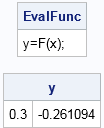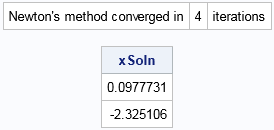A popular way to use lists in the SAS/IML language is to pack together several related matrices into a single data structure that can be passed to a function. Imagine that you have written an algorithm that requires a dozen different parameters. Historically, you would have to pass those parameters to the function by explicitly listing each argument. If you use lists (introduced in SAS/IML 14.2, which is SAS 9.4M4), you can pack all parameters into one list and pass that list into the function module where the individual items can be extracted as needed.

### Example: An algorithm that requires multiple parameters

To illustrate this list-passing technique, consider the algorithm for Newton's method, which is an iterative method for finding the roots of a nonlinear systems of equations. An implementation of Newton's method is included the SAS/IML documentation. Newton's method requires several arguments:

• The name of a module that evaluates the function whose roots are sought.
• The name of a module that evaluates the Jacobian matrix (the derivative of the function).
• An initial guess for the solution.
• The maximum number of iterations before the algorithm decides that the method is not converging.
• A convergence criterion that determines the accuracy of the solution.

The example in the documentation (which was written in the 1980s) uses global variables and hard-codes the names of functions and the convergence criteria. The example is meant to demonstrate the basic ideas, but is not reusable. You can make the example more flexible and robust by passing parameters to the module as shown in the next section.

### Create a list of parameters

Suppose that you define the modules F and DF to evaluate a multivariate function and the matrix of partial derivatives (the Jacobian matrix), respectively. The following modules evaluate the same mathematical function and derivatives that are used in the SAS/IML documentation:

```proc iml; /* Newton's method to solve for roots of the system of equations F(x1,x2) = [ x1+x2-x1*x2+2 ] = [ 0 ] [ x1*exp(-x2)-1 ] [ 0 ] */ /* 1. define a function F that evaluates a multivariate function */ start F(x); x1 = x; x2 = x; /* extract the values */ f = (x1+x2-x1*x2+2) // (x1*exp(-x2)-1); /* evaluate the function */ return ( f ); finish F;   /* 2. define a function DF that evaluates the Jacobian of F */ start DF(x); x1 = x; x2 = x; /* extract the values */ J = {. ., . .}; J[1,1] = 1-x2; J[1,2] = 1-x1; J[2,1] = exp(-x2); J[2,2] = -x1*exp(-x2); return ( J ); finish DF;```

As shown in a previous article, you can create a named list that contains the parameters for Newton's algorithm. In SAS/IML 14.3, you can create the list by specifying a sequence of name-value pairs:

```/* 3. list of NAME = VALUE pairs (syntax uses SAS/IML 14.3) */ args = [#"Func" = "F", /* name of module that evaluates the function */ #"Deriv" = "DF", /* name of module that evaluates the deivative */ #"x0" = {0.1, -2.0}, /* initial guess for solution */ #"MaxIters" = 100, /* maximum iterations */ #"ConvCrit" = 1e-6 ]; /* convergence criterion */```

In SAS/IML 14.3, you can use the list item operator (\$) to specify an item in a list. You can also get the name of the function and use CALL EXECUTE to evaluate the function at the initial guess, as follows:

```x = args\$"x0"; /* initial guess */ EvalFunc = "y=" + args\$"Func" + "(x);"; /* string for "y=F(x);" */ call execute(EvalFunc); /* evaluates function at x */ print EvalFunc, y;```This is a useful trick for evaluating a function by name. The next section uses this trick inside a module that implements Newton's method.

### Pass a list of parameters to and from a SAS/IML module

You can now implement Newton's method by defining a SAS/IML module that takes one argument, which is a list. Inside the module, the parameters are extracted from the list and used to evaluate the functions F and DF and to control the iteration and convergence of Newton's method, as follows:

```/* 4. Newton's method for solving the roots for F(x)=0. The argument to the module is a list. */ start Newton( L ); x = L\$"x0"; /* initial guess */ EvalFunc = "y=" + L\$"Func" + "(x);"; /* string for "y=F(x);" */ EvalDeriv = "D=" + L\$"Deriv" + "(x);"; /* string for "D=DF(x);" */   call execute(EvalFunc); /* evaluate F at starting values */ converged = (max(abs(y)) < L\$"ConvCrit"); /* 0 or 1 */ do iter = 1 to L\$"Maxiters" /* iterate until max iterations */ while( ^converged ); /* or until convergence */ call execute(EvalDeriv); /* evaluate derivatives D=DF(x) */ delta = -solve(D,y); /* solve for correction vector */ x = x+delta; /* update x with new approximation */ call execute(EvalFunc); /* evaluate the function y=F(x)*/ converged = (max(abs(y)) < L\$"ConvCrit"); end; /* you can return x or a list that summarizes the results */ result = [#"x" = x, /* approximate root (if converged) */ #"iterations" = iter, /* total number of iterations */ #"status" = converged]; /* 1 if root found; 0 otherwise */ return ( result ); finish Newton;   /* 5. call Newton's method and pass in a list of parameters */ soln = Newton( args ); xSoln = soln\$"x"; /* get root */ if soln\$"status" then /* check convergence statust */ print "Newton's method converged in " (soln\$"iterations") " iterations", xSoln; else print "Newton's method did not converge in " (soln\$"iterations") " iterations";```The Newton module returns a named list that has three items. The item named "status" is a binary value that indicates whether the method converged. If the method converged, the item named "x" contains the approximate root. The item named "iterations" contains the number of iterations of the algorithm.

In summary, you can use lists to create a structure that contains many parameters. You can pass the list to a SAS/IML module, which can extract and use the parameters. This enables you to simplify the calling syntax for user-defined modules that require many parameters.

For additional examples of using lists to pass heterogeneous data to SAS/IML modules, see "More Than Matrices: SAS/IML Software Supports New Data Structures" (Wicklin, 2017, pp. 11–12) and the SAS/IML documentation for lists.

Share